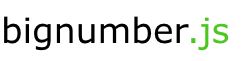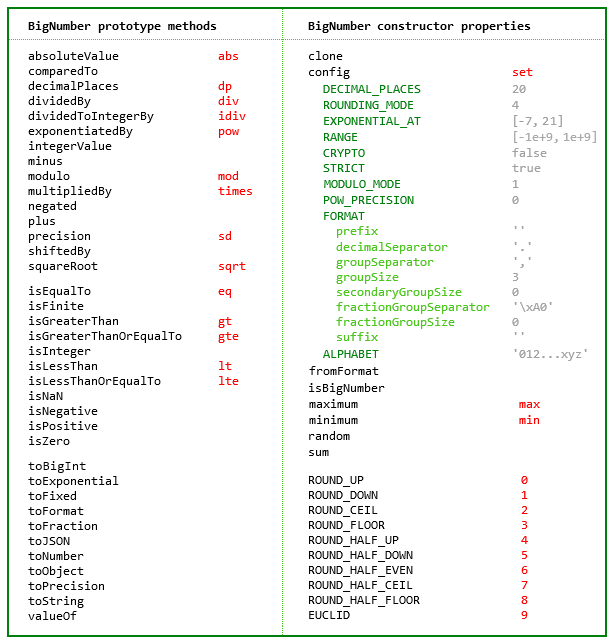# JavaScripting

• ×

## Bignumber.js

A JavaScript library for arbitrary-precision decimal and non-decimal arithmetic
Filed under  ›

• 🔾75%Overall
• 6,294
• 19.7 days
• 🕩776
• 👥18A JavaScript library for arbitrary-precision decimal and non-decimal arithmetic.

## Features

• Integers and decimals
• Simple API but full-featured
• Faster, smaller, and perhaps easier to use than JavaScript versions of Java's BigDecimal
• 8 KB minified and gzipped
• Replicates the `toExponential`, `toFixed`, `toPrecision` and `toString` methods of JavaScript's Number type
• Includes a `toFraction` and a correctly-rounded `squareRoot` method
• Supports cryptographically-secure pseudo-random number generation
• No dependencies
• Wide platform compatibility: uses JavaScript 1.5 (ECMAScript 3) features only
• Comprehensive documentation and test setIf a smaller and simpler library is required see big.js. It's less than half the size but only works with decimal numbers and only has half the methods. It also has fewer configuration options than this library, and does not allow `NaN` or `Infinity`.

See also decimal.js, which among other things adds support for non-integer powers, and performs all operations to a specified number of significant digits.

The library is the single JavaScript file bignumber.js or ES module bignumber.mjs.

### Browser

``````<script src='path/to/bignumber.js'></script>
``````

ES module

``````<script type="module">
import BigNumber from './path/to/bignumber.mjs';
``````

Get a minified version from a CDN:

``````<script src='https://cdn.jsdelivr.net/npm/bignumber.js@9.1.2/bignumber.min.js'></script>
``````

### Node.js

``````npm install bignumber.js
``````
``````const BigNumber = require('bignumber.js');
``````

ES module

``````import BigNumber from "bignumber.js";
import { BigNumber } from "./node_modules/bignumber.js/bignumber.mjs";
``````

### Deno

``````import BigNumber from 'https://raw.githubusercontent.com/mikemcl/bignumber.js/v9.1.2/bignumber.mjs';
import BigNumber from 'https://unpkg.com/bignumber.js@latest/bignumber.mjs';
``````

## Use

The library exports a single constructor function, `BigNumber`, which accepts a value of type Number, String or BigNumber,

``````let x = new BigNumber(123.4567);
let y = BigNumber('123456.7e-3');
let z = new BigNumber(x);
x.isEqualTo(y) && y.isEqualTo(z) && x.isEqualTo(z);      // true
``````

To get the string value of a BigNumber use `toString()` or `toFixed()`. Using `toFixed()` prevents exponential notation being returned, no matter how large or small the value.

``````let x = new BigNumber('1111222233334444555566');
x.toString();                       // "1.111222233334444555566e+21"
x.toFixed();                        // "1111222233334444555566"
``````

If the limited precision of Number values is not well understood, it is recommended to create BigNumbers from String values rather than Number values to avoid a potential loss of precision.

In all further examples below, `let`, semicolons and `toString` calls are not shown. If a commented-out value is in quotes it means `toString` has been called on the preceding expression.

``````// Precision loss from using numeric literals with more than 15 significant digits.
new BigNumber(1.0000000000000001)         // '1'
new BigNumber(88259496234518.57)          // '88259496234518.56'
new BigNumber(99999999999999999999)       // '100000000000000000000'

// Precision loss from using numeric literals outside the range of Number values.
new BigNumber(2e+308)                     // 'Infinity'
new BigNumber(1e-324)                     // '0'

// Precision loss from the unexpected result of arithmetic with Number values.
new BigNumber(0.7 + 0.1)                  // '0.7999999999999999'
``````

When creating a BigNumber from a Number, note that a BigNumber is created from a Number's decimal `toString()` value not from its underlying binary value. If the latter is required, then pass the Number's `toString(2)` value and specify base 2.

``````new BigNumber(Number.MAX_VALUE.toString(2), 2)
``````

BigNumbers can be created from values in bases from 2 to 36. See `ALPHABET` to extend this range.

``````a = new BigNumber(1011, 2)          // "11"
b = new BigNumber('zz.9', 36)       // "1295.25"
c = a.plus(b)                       // "1306.25"
``````

Performance is better if base 10 is NOT specified for decimal values. Only specify base 10 when you want to limit the number of decimal places of the input value to the current `DECIMAL_PLACES` setting.

A BigNumber is immutable in the sense that it is not changed by its methods.

``````0.3 - 0.1                           // 0.19999999999999998
x = new BigNumber(0.3)
x.minus(0.1)                        // "0.2"
x                                   // "0.3"
``````

The methods that return a BigNumber can be chained.

``````x.dividedBy(y).plus(z).times(9)
x.times('1.23456780123456789e+9').plus(9876.5432321).dividedBy('4444562598.111772').integerValue()
``````

Some of the longer method names have a shorter alias.

``````x.squareRoot().dividedBy(y).exponentiatedBy(3).isEqualTo(x.sqrt().div(y).pow(3))    // true
x.modulo(y).multipliedBy(z).eq(x.mod(y).times(z))                                   // true
``````

As with JavaScript's Number type, there are `toExponential`, `toFixed` and `toPrecision` methods.

``````x = new BigNumber(255.5)
x.toExponential(5)                  // "2.55500e+2"
x.toFixed(5)                        // "255.50000"
x.toPrecision(5)                    // "255.50"
x.toNumber()                        //  255.5
``````

A base can be specified for `toString`.

Performance is better if base 10 is NOT specified, i.e. use `toString()` not `toString(10)`. Only specify base 10 when you want to limit the number of decimal places of the string to the current `DECIMAL_PLACES` setting.

`````` x.toString(16)                     // "ff.8"
``````

There is a `toFormat` method which may be useful for internationalisation.

``````y = new BigNumber('1234567.898765')
y.toFormat(2)                       // "1,234,567.90"
``````

The maximum number of decimal places of the result of an operation involving division (i.e. a division, square root, base conversion or negative power operation) is set using the `set` or `config` method of the `BigNumber` constructor.

The other arithmetic operations always give the exact result.

``````BigNumber.set({ DECIMAL_PLACES: 10, ROUNDING_MODE: 4 })

x = new BigNumber(2)
y = new BigNumber(3)
z = x.dividedBy(y)                        // "0.6666666667"
z.squareRoot()                            // "0.8164965809"
z.exponentiatedBy(-3)                     // "3.3749999995"
z.toString(2)                             // "0.1010101011"
z.multipliedBy(z)                         // "0.44444444448888888889"
z.multipliedBy(z).decimalPlaces(10)       // "0.4444444445"
``````

There is a `toFraction` method with an optional maximum denominator argument

``````y = new BigNumber(355)
pi = y.dividedBy(113)               // "3.1415929204"
pi.toFraction()                     // [ "7853982301", "2500000000" ]
pi.toFraction(1000)                 // [ "355", "113" ]
``````

and `isNaN` and `isFinite` methods, as `NaN` and `Infinity` are valid `BigNumber` values.

``````x = new BigNumber(NaN)                                           // "NaN"
y = new BigNumber(Infinity)                                      // "Infinity"
x.isNaN() && !y.isNaN() && !x.isFinite() && !y.isFinite()        // true
``````

The value of a BigNumber is stored in a decimal floating point format in terms of a coefficient, exponent and sign.

``````x = new BigNumber(-123.456);
x.c                                 // [ 123, 45600000000000 ]  coefficient (i.e. significand)
x.e                                 // 2                        exponent
x.s                                 // -1                       sign
``````

For advanced usage, multiple BigNumber constructors can be created, each with its own independent configuration.

``````// Set DECIMAL_PLACES for the original BigNumber constructor
BigNumber.set({ DECIMAL_PLACES: 10 })

// Create another BigNumber constructor, optionally passing in a configuration object
BN = BigNumber.clone({ DECIMAL_PLACES: 5 })

x = new BigNumber(1)
y = new BN(1)

x.div(3)                            // '0.3333333333'
y.div(3)                            // '0.33333'
``````

To avoid having to call `toString` or `valueOf` on a BigNumber to get its value in the Node.js REPL or when using `console.log` use

``````BigNumber.prototype[require('util').inspect.custom] = BigNumber.prototype.valueOf;
``````

For further information see the API reference in the doc directory.

## Test

The test/modules directory contains the test scripts for each method.

The tests can be run with Node.js or a browser. For Node.js use

``````npm test
``````

or

``````node test/test
``````

To test a single method, use, for example

``````node test/methods/toFraction
``````

For the browser, open test/test.html.

## Minify

To minify using, for example, terser

``````npm install -g terser
``````
``````terser big.js -c -m -o big.min.js
``````

## Licence

The MIT Licence.

See LICENCE.